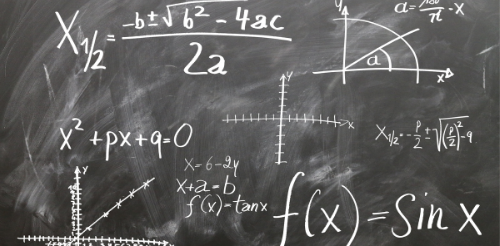## Mathematics

0(0 Ratings)

### Mathematics

Mathematics is the science of form, quantity and arrangement that deals with logic. In all we do, math is all around us. In our everyday lives, it is the building block for all, including mobile devices, architecture (old and modern), art, money, engineering, and even sports.

Mathematicians started to work with geometry as cultures grew, which computes areas and volumes to render angular measurements and has many practical applications. In everything from home construction to fashion and interior design, geometry is used.

### Math-Minor

GCSE, IGCSE, O level A level (all writers) (Math Makes Sense)

### Math-Major

(Pearson, McGraw Hill, Math Makes Sense, Foundation of Math, Applied Math’s, Nelson Math)

Nelson Math’s:

Grade 9 – Foundation of Mathematics (Applied) Mathematics

Grade 10 – Foundation of Mathematics (Applied) Mathematics

Grade 11 – Functions Mathematics Grade 11 – Functions and Applications Mathematics

Grade 11 – Foundation for College Mathematics Mathematics

Grade 11 – Mathematics for Work and Everyday Life Mathematics

Grade 12 – Calculus and Vectors Mathematics

Grade 12 – Mathematics of Data Management Mathematics

Grade 12 – Mathematics of College Technology Mathematics

Grade 12 – Foundations for College Mathematics Mathematics

Grade 12 – Mathematics for Work and Everyday Life Mathematics Pearson.

### For UK We Consider

Math makes sense grade 1 to 10

Math AS Level (Year 12)

Edexcel AS and A level Mathematics Pure Mathematics Year 1/AS Textbook

Edexcel AS and A level Mathematics Statistics & Mechanics Year 1/AS Textbook

Further Maths A-Level (Year 13)

Edexcel A level Further Mathematics Core Pure Mathematics Book 2

Grade 1 to 12: following all of the curriculum of UK.

### For USA We Follow

Math in Society By David Lippman Mathematical Discovery By Andrew M. Bruckner, Brian S. Thomson, Judith B. Bruckner

MacMillan/McGraw-Hill Houghton Mifflin Harcourt (Big Ideas Math)

Carnegie Learning

Pearson/Prentice Hall

### For AUSTRALIA The Grades Are

Grade 1 Mathematics, Pearson math’s make sense

Grade 2 Mathematics, Pearson math’s make sense

Grade 3 Mathematics, Pearson math’s make sense

Grade 4 Mathematics, Pearson math’s make sense

Grade 5 Mathematics, Pearson math’s make sense

Grade 6 Mathematics, Pearson math’s make sense

Grade 7 Cambridge Mathematics, Math’s Quest, Math’s Quest jacaranda, Pearson math’s, Essential math’s, Pearson math’s make sense

Grade 8 Mathematics Cambridge Mathematics, Math’s Quest, Math’s Quest jacaranda, Pearson math’s, Essential math’s

Grade 9: Math’s Quest, GCSE, IGCSE, and CAMBRIDGE CORE AND EXTENDED

Grade 9 – Foundation of Mathematics (Applied) Mathematics

Grade 10 – Foundation of Mathematics (Applied) Mathematics

Grade 11 – Functions and Applications Mathematics

Grade 11 – Foundation for College Mathematics Mathematics

Grade 11 – Mathematics for Work and Everyday Life Mathematics

Grade 12 – Calculus and Vectors Mathematics

Grade 12 – Mathematics of Data Management Mathematics

### For NEWZEALAND

NZ Year 1 (NCEA) Mathematics

NZ Year 2 (NCEA) Mathematics

NZ Year 3 (NCEA) Mathematics

NZ Year 4 (NCEA) Mathematics

NZ Year 5 (NCEA) Mathematics

NZ Year 6 (NCEA) Mathematics

NZ Year 7 (NCEA) Mathematics

NZ Year 8 (NCEA) Mathematics

NZ Year 9 (NCEA) Mathematics

NZ Year 10 (NCEA) Mathematics

NZ Year 11 (NCEA) Mathematics

NZ Year 12 (NCEA) Mathematics

NZ Year 13 (NCEA) Mathematics

#### Get a Free Trial.

We are waiting for you , Get a free trial class today.